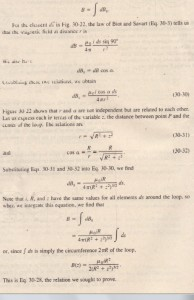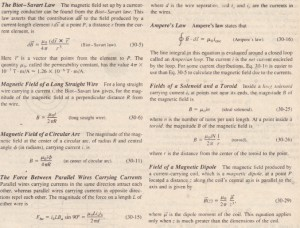# A Current Carrying Coil as a Magnetic Dipole

A Current-Carrying Coil as a Magnetic Dipole

So far we have examined the magnetic fields produced by current in a long straight wire, a solenoid, and a toroid, We turn our attention here to the field produced by a coil carrying a -current. You saw in Section 29-9 that such a coil behaves as a  magnetic dipole in that, if we place it in an external magnetic field B, a torque T given by

acts on it. Here jI is the magnetic dipole moment of the coil and has the negritude Nia, where N is the number of turns (or loops), i is the current in each turn. and A  is the area enclosed by each turn.Recall that the direction of jI is given by a curled-straight right-hand rule: Grasp the coil so that the fingers of your right hand curl around it in the direction
of the current; your extended thumb then points in the direction of the dipole moment jI.

Magnetic Field Of a Coil

We turn now to the other aspect of a current-carrying coil as a magnetic dipole. What magnetic field does it produce at a point in the surrounding space? The problem does not have enough symmetry to make Ampere’s law useful. so we must turn to the law of Biot and Savart. For simplicity, we first consider only a coil with a single circular loop and only points on its central axis, which we take to be a z axis. We shall show that the magnitude of the magnetic field at such points is

Proof Of Equation

the back half of a circular loop of radius R carrying a current’i. Consider a point P on the axis of the loop, a distance z from its plane. Let us apply the law of Biot and Savart to a differential element ds of the loop, located at the left side of the loop. The ‘length vector die for this element points perpendicularly out of the page. The angle (J between ds and r in  the plane formed by these two vectors is perpendicular to the plane of the figure and contains both brands. horn the law of Biot and Savart (and the right-hand rule), the differential field is produced at point P by the current   in this element is perpendicular to this plane and rush is directed in the plane of the figure, perpendicular to F, as indicated in  LeI us resolve dB into two components: dBIl along the axis of the loop and dB J. .ndiculur to this axis. From the symmetry, the vector sum of all the components dB J. due to all the loop elements ds is zero. This leaves only the axial components dBIl and we have.REVIEW & SUMMARY